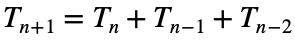# Problem 55985. Generate Tribonacci Sequence

The "Tribonacci" sequence is an extension of the idea of the Fibonacci sequence:That is, each new term is the sum of the three previous terms.
If the sequence starts with 0, 1, 1, the first 10 Tribonacci numbers are: 0, 1, 1, 2, 4, 7, 13, 24, 44, 81.
If the sequence instead starts with 2, 3, 7, the first 15 Tribonacci numbers are: 2, 3, 7, 12, 22, 41, 75, 138, 254, 467, 859, 1580, 2906, 5345, 9831.
Given a vector of three numbers, Tstart, and an integer n (> 3), return an n-element vector of the first n Tribonacci numbers starting with Tstart.
T = tribo([2 3 7],15)
T =
2 3 7 12 22 41 75 138 254 467 859 1580 2906 5345 9831

### Solution Stats

62.73% Correct | 37.27% Incorrect
Last Solution submitted on Nov 27, 2023

### Community Treasure Hunt

Find the treasures in MATLAB Central and discover how the community can help you!

Start Hunting!